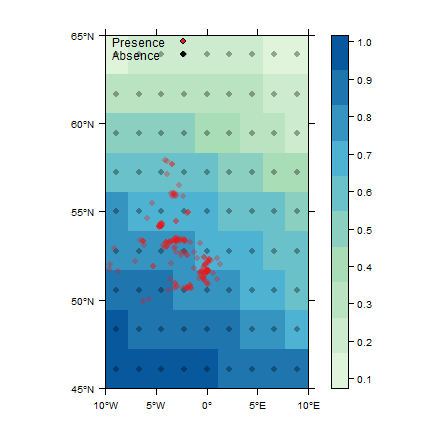# Using zoon modules interactively.

While the point of zoon is to run full workflows which are then reproducible, during development of modules it can be useful to run individual modules in the same way you would run normal R functions.

It is not entirely simple to do this, so this vignette just clarifies how.

First load packages. You need to explicitely load Dismo as we are now going to use Dismo functions outside the zoon environment.

library(dismo)
library(zoon)

This is the workflow we will run. It might be worth running it here to make sure there are no problems.

w <- workflow(UKAnophelesPlumbeus, UKAir, OneHundredBackground, LogisticRegression, PrintMap)
## Occurrence data does not have a "crs" column, zoon will assume it is in the same projection as the covariate data
## There are fewer than 100 cells in the environmental raster.
## Using all available cells (81) insteadIt’s worth noting that this is a simple workflow. Chaining modules will be fairly easy but depends on the module type. Workflows using list() are likely to not be easy.

Get the modules from the zoon repository and load them into the working environment.

LoadModule('UKAnophelesPlumbeus')
##  "UKAnophelesPlumbeus"
LoadModule('UKAir')
##  "UKAir"
LoadModule('OneHundredBackground')
##  "OneHundredBackground"
LoadModule('LogisticRegression')
##  "LogisticRegression"
LoadModule('PrintMap')
##  "PrintMap"

Run the data modules. To chain occurrence modules, just rbind the resulting dataframes. To chain covariate modules, use raster::stack to combine the covariate data.

oc <- UKAnophelesPlumbeus()
cov <- UKAir()

We have to run ExtractAndCombData. This combines the occurrence and raster data.

data <- ExtractAndCombData(oc, cov)
## Occurrence data does not have a "crs" column, zoon will assume it is in the same projection as the covariate data

Next run the process and model modules. To chain process models, simply run each in turn with the output of one going into the next. The simple way to run model modules is to use the module function as below. If crossvalidation is important then you need to run the modules slightly differently (see below).

proc <- OneHundredBackground(data)
## There are fewer than 100 cells in the environmental raster.
## Using all available cells (81) instead
mod <- LogisticRegression(proc$df) Finally, combine some output into a list and run the output modules. model <- list(model = mod, data = proc$df)

out <- PrintMap(model, cov)## Cross and external validation

Crossvalidation requires the modules to be run using the function RunModels which runs the model on each fold of the crossvalidating data and predicts the remaining data. It also runs a model and predicts any external validation data.

modCrossvalid <- RunModels(proc$df, 'LogisticRegression', list(), environment()) modelCrossvalid <- list(model = modCrossvalid$model, data = proc$df) out <- PrintMap(modelCrossvalid, proc$ras)## Running workflows with list.

As mentioned above, workflows using list() are likely to not be easy, but then these aren’t particularly required while developing a package. To run workflows using list, it would be best to use LoadModule as above and then run through the workflow source code interactively.